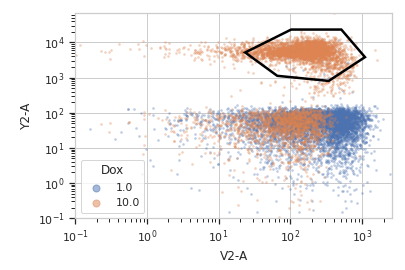# cytoflow.operations.polygon¶

class cytoflow.operations.polygon.PolygonOp[source]

Apply a polygon gate to a cytometry experiment.

name

The operation name. Used to name the new metadata field in the experiment that’s created by apply()

Type: Str
xchannel, ychannel

The names of the x and y channels to apply the gate.

Type: Str
xscale, yscale

The scales applied to the data before drawing the polygon.

Type: {‘linear’, ‘log’, ‘logicle’} (default = ‘linear’)
vertices

The polygon verticies. An ordered list of 2-tuples, representing the x and y coordinates of the vertices.

Type: List((Float, Float))

Notes

This module uses matplotlib.path.Path() to represent the polygon, because membership testing is very fast.

You can set the verticies by hand, I suppose, but it’s much easier to use the interactive view you get from default_view() to do so.

Examples

Make a little data set.

>>> import cytoflow as flow
>>> import_op = flow.ImportOp()
>>> import_op.tubes = [flow.Tube(file = "Plate01/RFP_Well_A3.fcs",
...                              conditions = {'Dox' : 10.0}),
...                    flow.Tube(file = "Plate01/CFP_Well_A4.fcs",
...                              conditions = {'Dox' : 1.0})]
>>> import_op.conditions = {'Dox' : 'float'}
>>> ex = import_op.apply()


Create and parameterize the operation.

>>> p = flow.PolygonOp(name = "Polygon",
...                    xchannel = "V2-A",
...                    ychannel = "Y2-A")
>>> p.vertices = [(23.411982294776319, 5158.7027015021222),
...               (102.22182270573683, 23124.058843387455),
...               (510.94519955277201, 23124.058843387455),
...               (1089.5215641232173, 3800.3424832180476),
...               (340.56382570202402, 801.98947404942271),
...               (65.42597937575897, 1119.3133482602157)]


Show the default view.

>>> df = p.default_view(huefacet = "Dox",
...                    xscale = 'log',
...                    yscale = 'log')

>>> df.plot(ex)Note

If you want to use the interactive default view in a Jupyter notebook, make sure you say %matplotlib notebook in the first cell (instead of %matplotlib inline or similar). Then call default_view() with interactive = True:

df = p.default_view(huefacet = "Dox",
xscale = 'log',
yscale = 'log',
interactive = True)
df.plot(ex)


Apply the gate, and show the result

>>> ex2 = p.apply(ex)
>>> ex2.data.groupby('Polygon').size()
Polygon
False    15875
True      4125
dtype: int64

apply(experiment)[source]

Applies the threshold to an experiment.

Parameters: experiment (Experiment) – the old Experiment to which this op is applied a new :class:’Experiment, the same as old_experiment but with a new column of type bool with the same as the operation name. The bool is True if the event’s measurement is within the polygon, and False otherwise. Experiment util.CytoflowOpError – if for some reason the operation can’t be applied to this experiment. The reason is in CytoflowOpError.args
default_view(**kwargs)[source]
class cytoflow.operations.polygon.PolygonSelection[source]

Plots, and lets the user interact with, a 2D polygon selection.

interactive

is this view interactive? Ie, can the user set the polygon verticies with mouse clicks?

Type: bool
xchannel, ychannel

The channels to use for this view’s X and Y axes. If you created the view using default_view(), this is already set.

Type: String
xscale, yscale

The way to scale the x axes. If you created the view using default_view(), this may be already set.

Type: {‘linear’, ‘log’, ‘logicle’}
op

The IOperation that this view is associated with. If you created the view using default_view(), this is already set.

Type: Instance(IOperation)
xlim, ylim

Set the min and max limits of the plots’ x and y axes.

Type: (float, float)
xfacet, yfacet

Set to one of the conditions in the Experiment, and a new row or column of subplots will be added for every unique value of that condition.

Type: String
huefacet

Set to one of the conditions in the in the Experiment, and a new color will be added to the plot for every unique value of that condition.

Type: String
huescale

How should the color scale for huefacet be scaled?

Type: {‘linear’, ‘log’, ‘logicle’}

Examples

In a Jupyter notebook with %matplotlib notebook

>>> s = flow.PolygonOp(xchannel = "V2-A",
...                    ychannel = "Y2-A")
>>> poly = s.default_view()
>>> poly.plot(ex2)
>>> poly.interactive = True

plot(experiment, **kwargs)[source]

Plot the scatter plot, and then plot the selection on top of it.

Parameters: experiment (Experiment) – The Experiment` to plot using this view. title (str) – Set the plot title xlabel, ylabel (str) – Set the X and Y axis labels huelabel (str) – Set the label for the hue facet (in the legend) legend (bool) – Plot a legend for the color or hue facet? Defaults to True. sharex, sharey (bool) – If there are multiple subplots, should they share axes? Defaults to True. row_order, col_order, hue_order (list) – Override the row/column/hue facet value order with the given list. If a value is not given in the ordering, it is not plotted. Defaults to a “natural ordering” of all the values. height (float) – The height of each row in inches. Default = 3.0 aspect (float) – The aspect ratio of each subplot. Default = 1.5 col_wrap (int) – If xfacet is set and yfacet is not set, you can “wrap” the subplots around so that they form a multi-row grid by setting col_wrap to the number of columns you want. sns_style ({“darkgrid”, “whitegrid”, “dark”, “white”, “ticks”}) – Which seaborn style to apply to the plot? Default is whitegrid. sns_context ({“paper”, “notebook”, “talk”, “poster”}) – Which seaborn context to use? Controls the scaling of plot elements such as tick labels and the legend. Default is talk. despine (Bool) – Remove the top and right axes from the plot? Default is True. min_quantile (float (>0.0 and <1.0, default = 0.001)) – Clip data that is less than this quantile. max_quantile (float (>0.0 and <1.0, default = 1.00)) – Clip data that is greater than this quantile. xlim, ylim ((float, float)) – Set the range of the plot’s axis. alpha (float (default = 0.25)) – The alpha blending value, between 0 (transparent) and 1 (opaque). s (int (default = 2)) – The size in points^2. marker (a matplotlib marker style, usually a string) – Specfies the glyph to draw for each point on the scatterplot. See matplotlib.markers for examples. Default: ‘o’